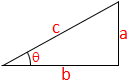# Cosine Calculator

The cosine (sine complement, Latin: cosinus, sinus complementi) of an angle is the ratio of the length of the adjacent side to the length of the hypotenuse, so called because it is the sine of the complementary or co-angle, the other non-right angle.cos = ?